# Kergin interpolation

Jump to: navigation, search

A form of interpolation providing a canonical polynomial of total degreewhich interpolates a sufficiently differentiable function at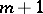points in. (Forand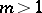there is no unique interpolating polynomial of degree.)

More specifically, givennot necessarily distinct points in,, andan-times continuously differentiable function on the convex hull of, the Kergin interpolating polynomialis of degree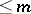and satisfies:

1)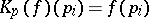for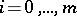; if a point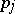is repeated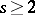times, then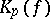andhave the same Taylor series up to order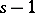at;

2) for any constant-coefficient partial differential operator (cf. also Differential equation, partial)of degree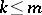, one has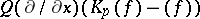is zero at some point of the convex hull of any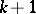of the points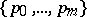; furthermore, ifsatisfies an equation of the form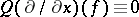, then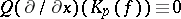;

3) for any affine mapping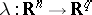(cf. also Affine morphism) andan-times continuously differentiable function on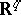one has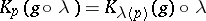, where;

4) the mapping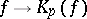is linear and continuous.

(In fact, 3)–4) already characterize the Kergin interpolating polynomial.)

The existence ofwas established by P. Kergin in 1980 [a2]. For,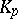reduces to Lagrange–Hermite interpolation (cf. also Hermite interpolation formula; Lagrange interpolation formula).

An explicit formula forwas given by P. Milman and C. Micchelli [a3]. The formula shows that the coefficients ofare given by integrating derivatives ofover faces in the convex hull of. More specifically, let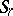denote the simplex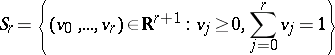and use the notation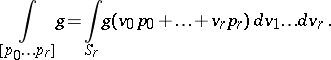Then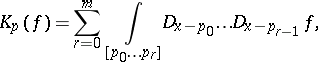where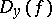denotes the directional derivative ofin the direction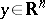.

Kergin interpolation also carries over to the complex case (as does Lagrange–Hermite interpolation), as follows. Let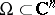be a-convex domain (i.e. every intersection ofwith a complex affine line is connected and simply connected, cf. also-convexity) and let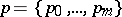bepoints in. Forholomorphic onthere is a canonical analytic interpolating polynomial,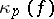, of total degreethat satisfies properties corresponding to 1), 3), 4) above. Ifis convex (identifyingwith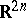), then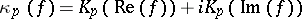. For general-convex domains (i.e. not necessarily real-convex), the formula for, due to M. Andersson and M. Passare [a1], is analogous to the Milman–Micchelli formula above, but uses integration over singular chains.

There is a generalization of the Hermite remainder formula for Kergin interpolation ifis a bounded-convex domain with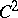defining functionandholomorphic inand continuous up to the boundaryof[a1]. It is: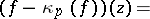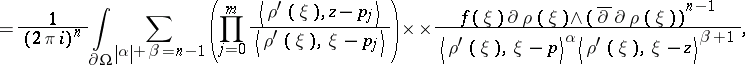whereis anmulti-index,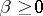is an integer,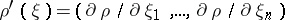for,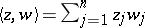, and.